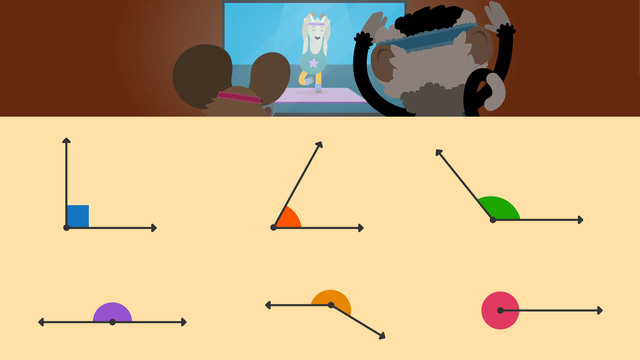# Types of Angles

Content Types of AnglesRating

Ø 5.0 / 4 ratings
The authorsTeam Digital
Types of Angles
CCSS.MATH.CONTENT.4.G.A.1

### TranscriptTypes of Angles

Nico and Nia are ecstatic to take their first yoga class! However, it appears that they need to work on their balance. In order for them to understand how to do the poses, they must first learn about the different... Types of Angles. An angle is formed when two rays intersect and meet at the same point. We call this point the VERTEX. Angles are named based on the size of the angle opening, which is known as the arc. Here are some examples of angles used in geometry. The right angle is the most common and easiest to identify. Right angles, also known as ninety-degree angles, are identified by the square they form in the corner of the arc. Acute angles are characterized by a small arc opening. Acute angles are those that are less than ninety degrees. Obtuse angles have a large opening with a wide arc. Obtuse angles are those that are greater than ninety degrees but less than one hundred eighty degrees. When two rays extend from the vertex in opposite directions, they form a straight angle or line. A straight angle measures EXACTLY one-hundred-eighty degrees. A reflex angle is formed when the arc of an angle exceeds that of a straight line. These angles have a value greater than one hundred-eighty degrees but less than three hundred sixty degrees. When an angle completes a full rotation, it is said to be a complete angle. This angle has three hundred-sixty degrees and is commonly referred to as a full circle. Now let's classify some angles based on their characteristics. Is this a right, acute, or obtuse angle? This angle has an opening that is less than ninety degrees, so it is an acute angle. Is this a right, acute, or obtuse angle? The arc is a ninety-degree angle and creates a square, it is a right angle. Is this a right, obtuse, or reflex angle? This is a reflex angle because it is greater than one hundred eighty degrees. Time for one more. Is this an acute, obtuse, or reflex angle? This angle is obtuse because it is wider than ninety degrees, but less than one hundred eighty degrees. Before we get back to yoga class, let's review. Remember... An angle is formed when two rays intersect and meet at the same point. Angles are named based on the size of the angle opening, which is known as the arc. Looks like Nico and Nia are really starting to get the hang of things!

## Types of Angles exercise

Would you like to apply the knowledge you’ve learned? You can review and practice it with the tasks for the video Types of Angles .
• ### Identify the types of angles.

Hints

A right angle is the most common angle and forms a perfect square at the corner of the arc.

A complete circle is 360°.

An acute angle is less than 90°.

An obtuse angle is more than 90° and less than 180°.

Solution
• A straight angle or line is 180°.
• A reflex angle is more than 180° but less than 360°.
• A right angle is 90°.
• A complete angle is 360°, or a full circle.
• ### Define the terms.

Hints

Most angles have arcs.

When comparing types of angles, think of one as cute and small. This angle is an acute angle.

A vertex is where two or more lines meet.

Solution
• The vertex is the point where two rays meet to form an angle.
• The arc is the size of the angle opening.
• Acute angles have a small arc less than 90°.
• Obtuse angles have a large arc, more than 90° and less than 180°.
• ### Sort different angles.

Hints

An acute angle is less than 90°.

An obtuse angle is more than 90° and less than 180°.

Two choicies are acute angles, two choices are obtuse angles, and one choice is a right angle.

Solution

OBTUSE ANGLES are more than 90° and less than 180°

• 120°
• 150°
ACUTE ANGLES are less than 90°
• 60°
• 45°
A RIGHT ANGLE is 90°

• ### Identify the types of angles.

Hints

A complete circle is 360°.

An acute angle is less than 90°.

A reflex angle is more than 180° but less than 360°.

A straight angle is also known as a line.

Solution

REFLEX ANGLES are more than 180° and less than 360°

• 215°
• 197°
ACUTE ANGLES are less than 90°
• 45°
A STRAIGHT ANGLE is 180°
A COMPLETE ANGLE is 360°, or a full circle

• ### Label the angle.

Hints

An acute angle has a small arc of less than 90°.

An obtuse angle has a large arc of more than than 90° and less than 180°.

A complete angle is 360°

Solution

A right angle is the most common angle and forms a perfect square at the corner of the arc. Its arc is 90°

• ### Name and define the angles.

Hints

This is an obtuse angle because it is greater than 90° and less than 180°.

A straight angle is also known as a line.

A complete circle is 360°.

An acute angle can be thought of as cute and small.

Solution
• A straight angle is 180°
• A right angle is 90°
• A reflex angle is greater than 180° and less than 360°
• An acute angle is less than 90°
• A complete angle is 360°
• An obtuse angle is greater than 90° and less than 180°